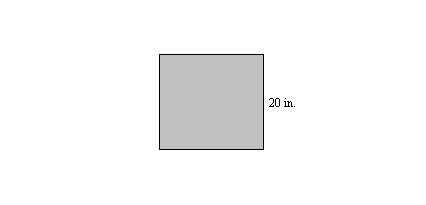Name:    Quiz 4.4 - 4.5

Multiple Choice
Identify the choice that best completes the statement or answers the question.

Find the percent of the number.

1.

20% of 70
 a. 14 c. 56 b. 10 d. 1400

2.

58% of 91
 a. 56.61 c. 38.22 b. 52.78 d. 50.54

3.

6% of 70
 a. 4.7 c. 5.6 b. 4.2 d. 65.8

4.

270% of 50
 a. 185 c. 135 b. 136 d. 134

5.

245% of 56
 a. 193.2 c. 137.2 b. 138.1 d. 134.1

6.

7.5% of 80
 a. 2.4 c. 4.51 b. 6 d. 60

7.

0.6% of 30
 a. 0.018 c. 18 b. 1.8 d. 0.18

Estimate the percent of the number.

8.

33% of 53
 a. 15 c. 30 b. 150 d. 7

9.

4% of 20
 a. 3 c. 0 b. 10 d. 1

10.

54% of 396
 a. 2000 c. 200 b. 100 d. 400

11.

61% of 1800
 a. 2,160 c. 10,800 b. 540 d. 1,080

12.

A book costs \$10 at one book store. About how much more does the book cost when the book store increases its prices 27%?
 a. \$6 c. \$13 b. \$1 d. \$3

Use compatible numbers to estimate the percent of the number.

13.

26% of 61
 a. 45 c. 30 b. 7 d. 15

Numeric Response

1.

A new pair of shoes is advertised for \$70. The sales tax is 7%. What is the total cost of the shoes?

2.

How many minutes is 60% of 2 hours?

1.

If 60 is 150% of a number, is the number greater or less than 60?

2.

Write a real-world problem that can be solved by estimating 6% of 170.

Other

1.

Extended Response  The length of a rectangle is 110% of its width.a.   What is the area of the rectangle?
b.   The width of the rectangle is halved. What percent of the width is the length now? Explain your reasoning.